## Frequently asked questions

### Open Channel Flow

For open channel flow use the Chezy-Manning equation:Q = Flow Rate (cfs)

n = Roughness Coefficient

A = Area of Water

R = Hydraulic Radius

S = Slope (decimal form)

The hydraulic radius is the area of water divided by the wetted perimeter which is the perimeter of the sides of the channel which are in contact with water.

### Stormwater Collection

There are many components used in the collection of stormwater some examples include:

Culverts: A pipe carrying water under or through a feature. Culverts often carry brooks or creeks under roadways. Culverts must be designed for large intensity storm events.

Stormwater Inlets: Roadside storm drains which collect water from gutter flow or roadside swales.

Gutter/Street flow: Flow which travels along the length of the street or gutter.

Storm Sewer Pipes: Pipes installed under the road which carry the water from inlets to a suitable outlet.

The principle of Conservation of Flow is often applicable when analyzing drainage. It states that the flow in must equal a flow out and therefore:

Q1 + Q2 = Q3

### Storm Characteristics

Storm characteristics include duration, total volume, and intensity

Duration: The length of time of a storm. Often measured in days and hours.

Total Volume: The entire amount of precipitation throughout the duration of the storm in a defined area.

Storm Intensity: Total volume of the storm divided by the duration of the storm event. Intensities can be averaged over the entire storm or at shorter intervals to provide instantaneous high intensity portions of the storm. Hyetographs are bar graphs used to measure instantaneous rainfall intensities over time.

A design storm must be specified when performing any calculations. The design storm is defined by its recurrence interval which is the given amount of time it is likely to see a storm of a certain intensity. Design storms are often 10, 20, 50, or 100-year storms meaning a storm of a certain intensity would only occur once within the given duration.

Hyetographs – Graphical representation of rainfall distribution over time

Hydrograph – Graphical representation of rate of flow vs time past a given point often in a river, channel or conduit

Parts of a Hydrograph are shown graphically:### Runoff Analysis

The rational method can be used to determine the flow rate from runoff of a drainage area. The equation is:

Q = ACi

Q = Flow Rate (cfs)

A = Drainage Area (Acres)

C = Runoff Coefficient

i = Rainfall Intensity (in/hr)

For total flow from multiple areas to a single outlet the conservation of flow principle is applied and the total is the sum of all flow into the outlet.

Time of Concentration, tc: The time of travel for water to move from the hydraulically most remote point in a watershed to the outlet. The time of concentration is the sum of three components:

tc=tsheet+tshallow+tchannel

For approximately the first 300 ft, water moves as sheet flow: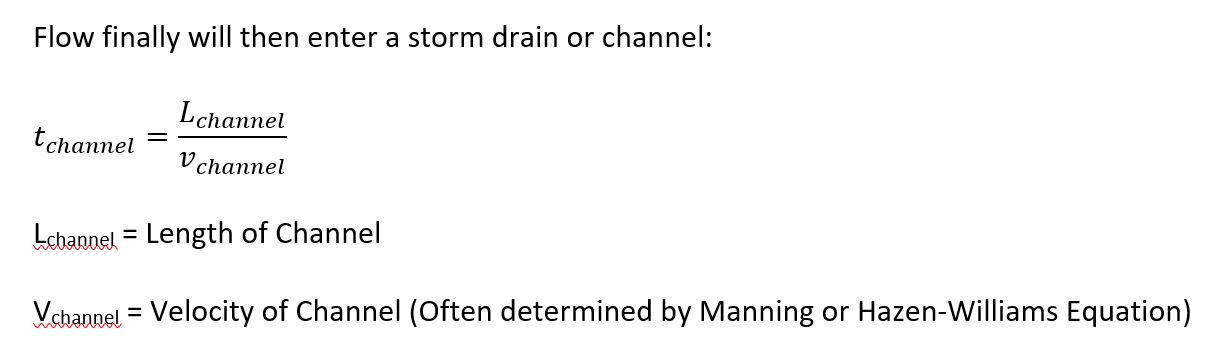### NRCS/SCS Runoff Method

This is an alternative method for determining runoff: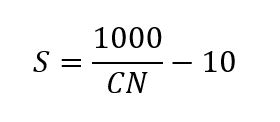S = Storage Capacity of Soil (in.)

CN = NRCS Curve Number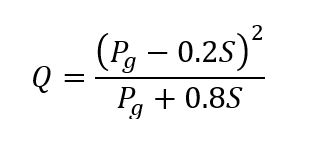Q = Runoff (in.)

Pg = Gross Rain Fall (in.)

### Detention and Retention Ponds

Detention and retention ponds are often used to collect water for flood control and stormwater runoff treatment.

Detention Ponds: Also known as dry ponds. These are ponds which are often kept dry except during flood events. The pond will fill up during increased precipitation to control the flow intensity. This is common in dry, arid or urban areas to prevent excessive flooding. The ponds typically will be designed to hold water for about 24 hours. Detention ponds also controls the amount of sediment since it is captured in the pond and then typically becomes accessible after the pond has dried.

Retention Ponds: Also called wet ponds since they contain a volume of water at all times. The elevation of the water will vary depending on precipitation but will always maintain a permanent amount of water based on low flow conditions. This allows sediment control since the deposits will settle to the bottom and allow for collection.

### Pressure Conduits

The Darcy Equation is used for fully turbulent flow to find the head loss due to friction. The equation is:hf = Head Loss Due to Friction (ft)

f = Darcy Friction Factor

L = Length of Pipe (ft)

v = Velocity of Flow (ft/sec)

D = Diameter of Pipe (ft)

g = Acceleration Due to Gravity, Use 32.2 ft/sec2

The Hazen-Williams equation is also used to determine head loss due to friction. Be aware of units as this equation may be presented in different forms. The most common is the following: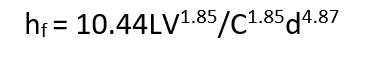hf = Head Loss due to Friction (ft)

L = Length (ft)

V = Velocity (gallons per minute)

C = Roughness Coefficient

d = Diameter (ft)

In addition to these losses, there is also what is called minor losses of energy due to friction

Minor Losses – Friction losses due to fittings in the line, changes in the dimensions of the pipe, or changes in direction

• Minor losses can be calculated as per the Method of Loss coefficients.
• Each change in the flow of pipe is assigned a loss coefficient, K
• Loss coefficients for fittings are most often determined and provided by the manufacturer
• Loss coefficients for sudden changes in area must be determined:

For Sudden Expansions: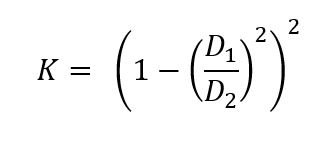For Sudden Contractions:• D1=Smaller diamter pipe

Loss coefficients are then multiplied by the kinetic energy to determine the loss.### Bernoulli Energy Equation

The Bernoulli equation for the conservation of energy states that the total energy is equal to the sum of the pressure + kinetic energy + potential energy and is conserved at any point in the system. Therefore:Epr = Pressure = p

Ev = Kinetic Energy = v2/2g

v = Velocity (ft/s)

g = Acceleration Due to Gravity (32.2 ft/s2)

Ep = Potential Energy = z = Height above point of interest to surface of water (ft)